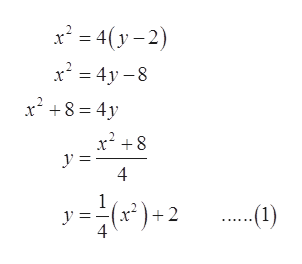# For Exercise, graph the equations and functions.x2 = 4(y − 2)

Question
2 views

For Exercise, graph the equations and functions.

x2 = 4(y − 2)

check_circle

Step 1

To sketch the graph of the given equation.

Step 2

Given information:

Equation is shown below.

Step 3

Calculation:help_outlineImage Transcriptionclosex' = 4(y – 2) x = 4y – 8 x² +8 = 4y x' +8 4 y =- x)+ 2 .(1) fullscreen

### Want to see the full answer?

See Solution

#### Want to see this answer and more?

Solutions are written by subject experts who are available 24/7. Questions are typically answered within 1 hour.*

See Solution
*Response times may vary by subject and question.
Tagged in

### Equations and In-equations